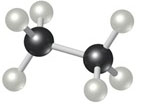# Problem: Calculate the percentage of carbon by mass in each of the compounds represented by the following models. (Carbon atoms are black spheres, hydrogen are grey, oxygen are red.)

###### FREE Expert Solution

Mass percent:

Compound is: CH3—H3C or C2H6

• Molar mass C2H6 = 30.07 g/mol
92% (99 ratings)###### Problem Details

Calculate the percentage of carbon by mass in each of the compounds represented by the following models. (Carbon atoms are black spheres, hydrogen are grey, oxygen are red.)Frequently Asked Questions

What scientific concept do you need to know in order to solve this problem?

Our tutors have indicated that to solve this problem you will need to apply the Mass Percent concept. You can view video lessons to learn Mass Percent. Or if you need more Mass Percent practice, you can also practice Mass Percent practice problems.

What professor is this problem relevant for?

Based on our data, we think this problem is relevant for Professor Ingram's class at UWATERLOO.